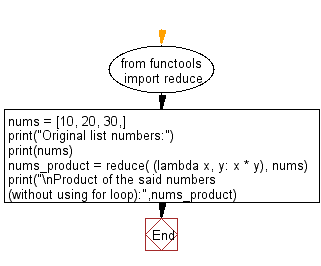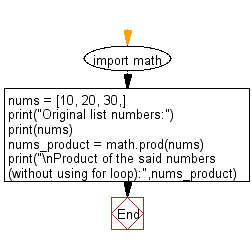﻿ Python: Compute the product of a list of integers - w3resource# Python: Compute the product of a list of integers

## Python Basic: Exercise-115 with Solution

Write a Python program to compute the product of a list of integers (without using for loop).

Sample Solution-1:

Python Code:

``````from functools import reduce
nums = [10, 20, 30,]
print("Original list numbers:")
print(nums)
nums_product = reduce( (lambda x, y: x * y), nums)
print("\nProduct of the said numbers (without using for loop):",nums_product)
```
```

Sample Output:

```Original list numbers:
[10, 20, 30]
Product of the numbers :  6000
```

Flowchart:## Visualize Python code execution:

The following tool visualize what the computer is doing step-by-step as it executes the said program:

Sample Solution-2:

Python Code:

``````import math
# Python version 3.9.
nums = [10, 20, 30,]
print("Original list numbers:")
print(nums)
nums_product = math.prod(nums)
print("\nProduct of the said numbers (without using for loop):",nums_product)
```
```

Sample Output:

```Original list numbers:
[10, 20, 30]
Product of the numbers :  6000
```

Flowchart:## Visualize Python code execution:

The following tool visualize what the computer is doing step-by-step as it executes the said program:

Python Code Editor:

Have another way to solve this solution? Contribute your code (and comments) through Disqus.

What is the difficulty level of this exercise?

Test your Python skills with w3resource's quiz

﻿

## Python: Tips of the Day

Try-catch-else construct:

```try:
foo()
except Exception:
print("Exception occured")
else:
print("Exception didnt occur")
finally:
print("Always gets here")
```